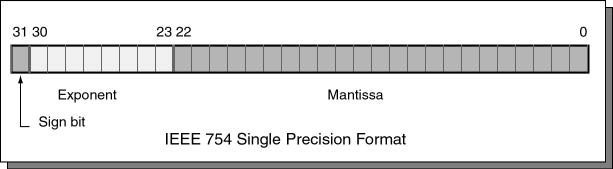The exponent.

# The ExponentThe eight bits 23 through 30 contain the exponent. The exponent is an integer, but may be negative, zero, or positive. You might guess that an 8-bit two's complement integer would work perfectly for this, but a different type of notation is used.

The exponent is expressed using a biased integer. The true exponent is added to `+12710` resulting in an unsigned binary integer. The biased integer is encoded using 8-bit unsigned binary.

• A biased exponent of `+127` represents the actual exponent 0.
• A biased exponent of `+128` represents the actual exponent 1.
• A biased exponent of `+126` represents the actual exponent -1.

Exceptions: (1) A floating point value of `0.0` is represented with a mantissa of 23 zero bits and an exponent of 8 zero bits. It is not legal to have an exponent of zero with a non-zero mantissa. (2) The exponent `25510` `(1111 1111)` is used to signal various problems, such as division by zero.

### QUESTION 15:

What is the mantissa when the entire float is zero?